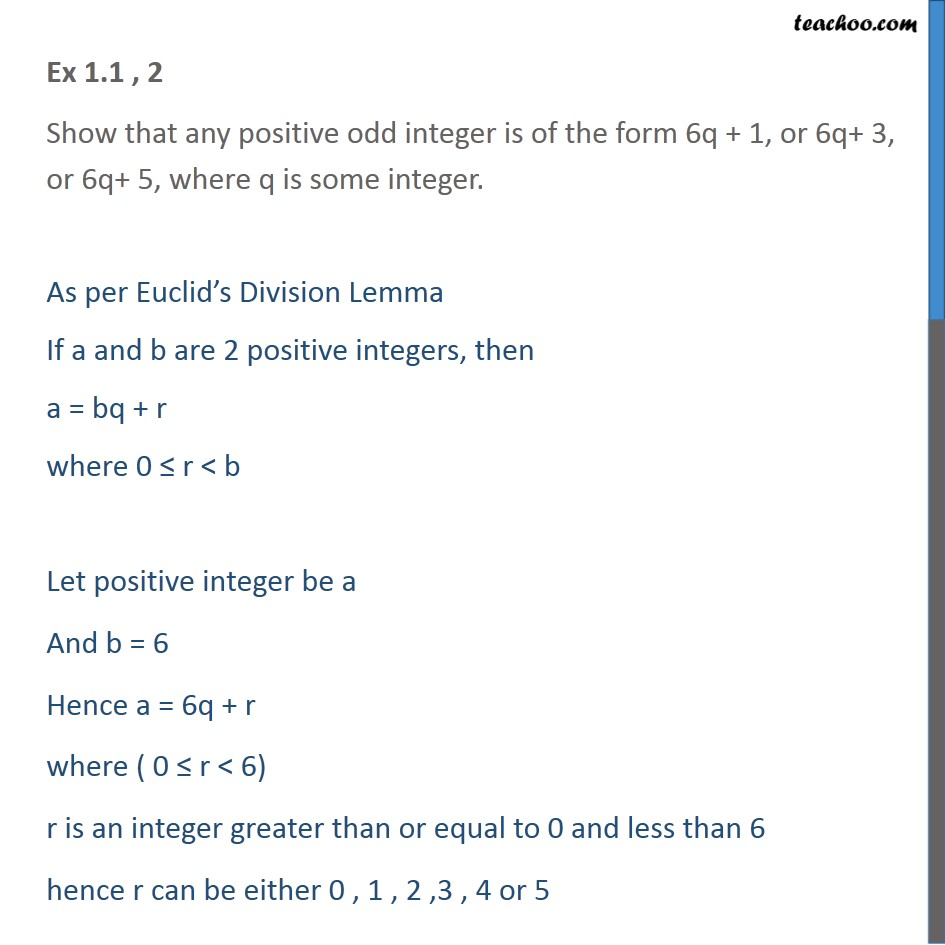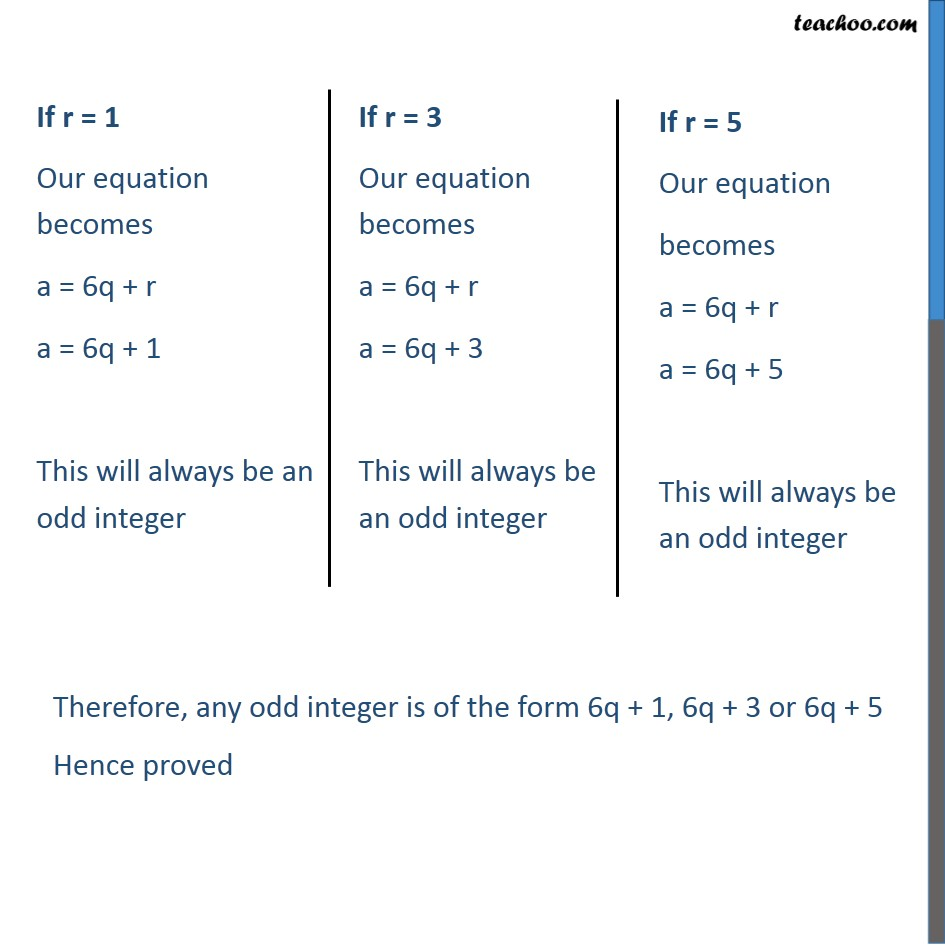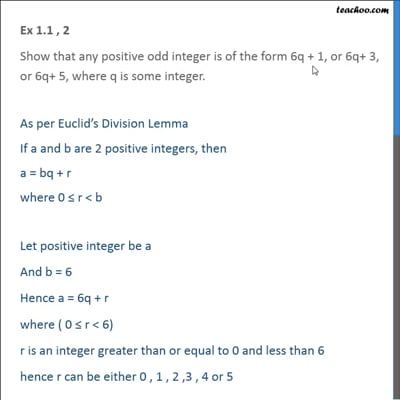Ex 1.1

Chapter 1 Class 10 Real Numbers
Serial order wiseThis video is only available for Teachoo black users

Maths Crash Course - Live lectures + all videos + Real time Doubt solving!

### Transcript

Ex 1.1 , 2 Show that any positive odd integer is of the form 6q + 1, or 6q+ 3, or 6q+ 5, where q is some integer. As per Euclid’s Division Lemma If a and b are 2 positive integers, then a = bq + r where 0 ≤ r < b Let positive integer be a And b = 6 Hence a = 6q + r where ( 0 ≤ r < 6) r is an integer greater than or equal to 0 and less than 6 hence r can be either 0 , 1 , 2 ,3 , 4 or 5 Therefore, any odd integer is of the form 6q + 1, 6q + 3 or 6q + 5 Hence proved